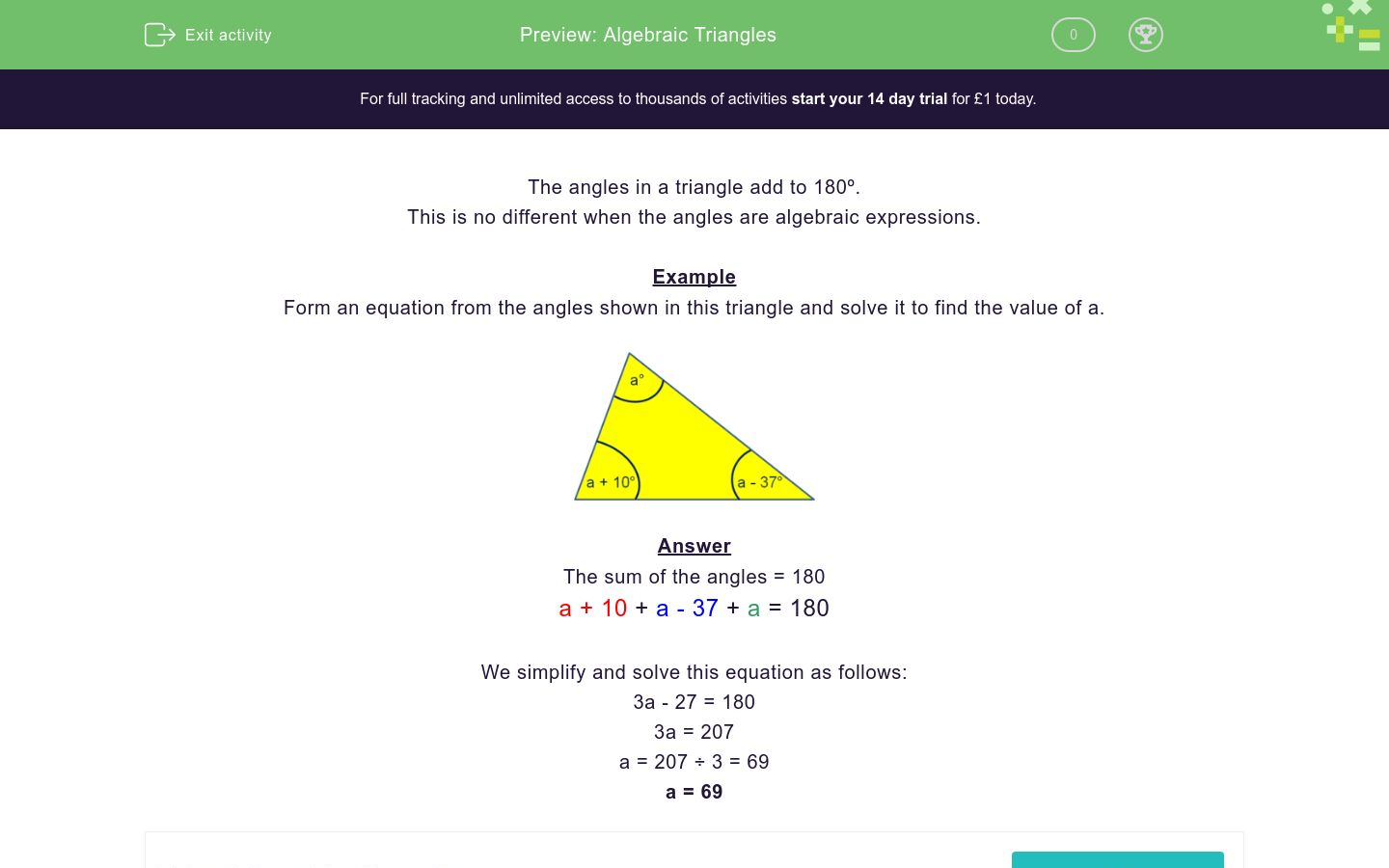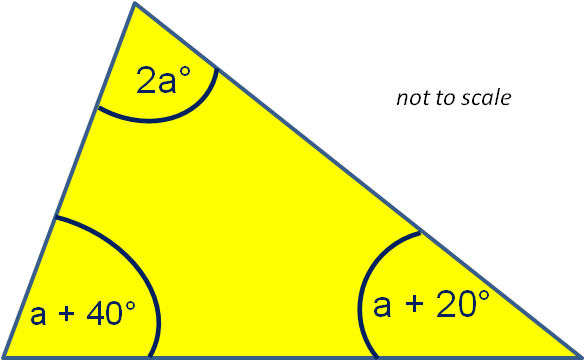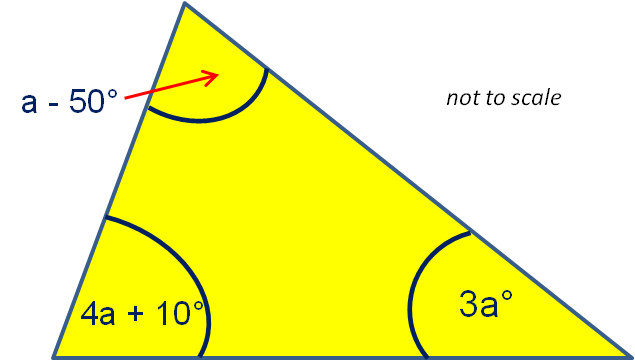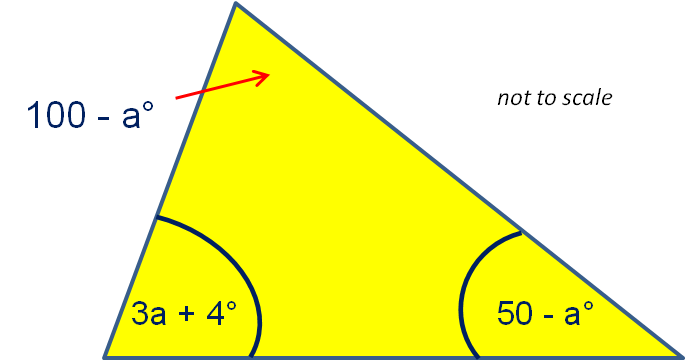# Algebraic Triangles

In this worksheet, students form and solve equations by adding together the algebraic angles shown in each triangle.Key stage:  KS 3

Curriculum topic:   Geometry and Measures

Curriculum subtopic:   Interpret Mathematical Relationships Algebraically/Geometrically

Difficulty level:### QUESTION 1 of 10

The angles in a triangle add to 180º.

This is no different when the angles are algebraic expressions.

Example

Form an equation from the angles shown in this triangle and solve it to find the value of a.The sum of the angles = 180

a + 10 + a - 37 + a = 180

We simplify and solve this equation as follows:

3a - 27 = 180

3a = 207

a = 207 ÷ 3 = 69

a = 69

Form an equation from the angles shown in this triangle and solve it to find the value of a.

(Just write the number)Form an equation from the angles shown in this triangle and solve it to find the value of a.

(Just write the number)Form an equation from the angles shown in this triangle and solve it to find the value of a.

(Just write the number)Form an equation from the angles shown in this triangle and solve it to find the value of a.

(Just write the number)Form an equation from the angles shown in this triangle and solve it to find the value of a.

(Just write the number)Form an equation from the angles shown in this triangle and solve it to find the value of a.

(Just write the number)Form an equation from the angles shown in this triangle and solve it to find the value of a.

(Just write the number)Form an equation from the angles shown in this triangle and solve it to find the value of a.

(Just write the number)Form an equation from the angles shown in this triangle and solve it to find the value of a.

(Just write the number)Form an equation from the angles shown in this triangle and solve it to find the value of a.

(Just write the number)• Question 1

Form an equation from the angles shown in this triangle and solve it to find the value of a.

(Just write the number)20
EDDIE SAYS
3a + 4a + 2a = 180 9a = 180
• Question 2

Form an equation from the angles shown in this triangle and solve it to find the value of a.

(Just write the number)22
EDDIE SAYS
3a + 3a - 50 + 4a + 10 = 180 10a - 40 = 180
• Question 3

Form an equation from the angles shown in this triangle and solve it to find the value of a.

(Just write the number)35
EDDIE SAYS
4a - 65 + 2a + 10 + a -10 = 180 7a - 65 = 180
• Question 4

Form an equation from the angles shown in this triangle and solve it to find the value of a.

(Just write the number)30
EDDIE SAYS
2a + a + 40 + a + 20 = 180 4a + 60 = 180
• Question 5

Form an equation from the angles shown in this triangle and solve it to find the value of a.

(Just write the number)20
EDDIE SAYS
60 - a + 3a + 30 + 4a - 30 = 180 6a + 60 = 180
• Question 6

Form an equation from the angles shown in this triangle and solve it to find the value of a.

(Just write the number)11
EDDIE SAYS
5a + 3a + 30 + 2a + 40 = 180 10a + 70 = 180
• Question 7

Form an equation from the angles shown in this triangle and solve it to find the value of a.

(Just write the number)27.5
EDDIE SAYS
a - 50 + 4a + 10 + 3a = 180 8a - 40 = 180
• Question 8

Form an equation from the angles shown in this triangle and solve it to find the value of a.

(Just write the number)25
EDDIE SAYS
2a - 50 + 3a + 5 + 4a = 180 9a - 45 = 180
• Question 9

Form an equation from the angles shown in this triangle and solve it to find the value of a.

(Just write the number)21
EDDIE SAYS
4a - 50 + 3a + 4 + 100 - a = 180 6a + 54 = 180
• Question 10

Form an equation from the angles shown in this triangle and solve it to find the value of a.

(Just write the number)26
EDDIE SAYS
100 - a + 3a + 4 + 50 - a = 180 a + 154 = 180
---- OR ----

Sign up for a £1 trial so you can track and measure your child's progress on this activity.

### What is EdPlace?

We're your National Curriculum aligned online education content provider helping each child succeed in English, maths and science from year 1 to GCSE. With an EdPlace account you’ll be able to track and measure progress, helping each child achieve their best. We build confidence and attainment by personalising each child’s learning at a level that suits them.

Get started